# How to Calculate and Solve for Distance Covered | Motion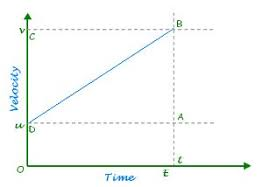The image above represents distance covered.

To compute for distance covered, three essential parameters are needed and these parameters are initial velocity (u), acceleration (a) and time (t).

The formula for calculating distance covered:

S = ut + 0.5at²

Where;

S = Distance Covered
u = Initial Velocity
t = Time
a = Acceleration

Let’s solve an example;
Find the distance covered when the initial velocity is 21, acceleration is 10 and the time is 15.

This implies that;

u = Initial Velocity = 21
t = Time = 15
a = Acceleration = 10

S = ut + 0.5at²
S = (21 x 15) + (0.5 x 10 x 15²)
S = 315 + (0.5 x 10 x 225)
S = 315 + (1125)
S = 1440

Therefore, the distance covered is 1440 m .

Calculating the Initial Velocity when the Distance Covered, the Acceleration and the Time is Given.

u = S – 0.5at2 / t

Where;

u = Initial Velocity
S = Distance Covered
t = Time
a = Acceleration

Let’s solve an example;
Find the initial velocity when the distance covered is 48 with a time of 8 and an acceleration of 9.

This implies that;

S = Distance Covered = 48
t = Time = 8
a = Acceleration = 9

u = S – 0.5at2 / t
u = 48 – 0.5(9)(82) / 8
u = 48 – 0.5(9)(64) / 8
u = 48 – 288 / 8
u = – 240 / 8
u = – 30

Therefore, the initial velocity is – 30 m/s.

Calculating the Time when the Distance Covered, the Initial Velocity and the Acceleration is Given.

t = S – u / 0.5a

Where;

t = Time
S = Distance Covered
u = Initial Velocity
a = Acceleration

Let’s solve an example;
Given that the distance covered is 52, the initial velocity is 22 and the acceleration is 15. Find the time?

This implies that;

S = Distance Covered = 52
u = Initial Velocity = 22
a = Acceleration = 15

t = S – u / 0.5a
t = 52 – 22 / 0.5(15)
t = 30 / 7.5
t = 4

Therefore, the time is 4 s.

Calculating the Acceleration when the Distance Covered, the Initial Velocity and the Time is Given.

a = S – ut / 0.5t2

Where;

a = Acceleration
S = Distance Covered
u = Initial Velocity
t = Time

Let’s solve an example;
Find the acceleration when the distance covered is 61, the initial velocity is 12 and the time is 5.

This implies that;

S = Distance Covered = 61
u = Initial Velocity = 12
t = Time = 5

a = S – ut / 0.5t2
a = 61 – (12 x 5) / 0.5(52)
a = 61 – 60 / 0.5(25)
a = 1 / 12.5
a = 0.08

Therefore, the acceleration is 0.08.

Nickzom Calculator – The Calculator Encyclopedia is capable of calculating the distance covered.

To get the answer and workings of the distance covered using the Nickzom Calculator – The Calculator Encyclopedia. First, you need to obtain the app.

You can get this app via any of these means:

To get access to the professional version via web, you need to register and subscribe for NGN 1,500 per annum to have utter access to all functionalities.
You can also try the demo version via https://www.nickzom.org/calculator

Apple (Paid) – https://itunes.apple.com/us/app/nickzom-calculator/id1331162702?mt=8
Once, you have obtained the calculator encyclopedia app, proceed to the Calculator Map, then click on Motion under Physics.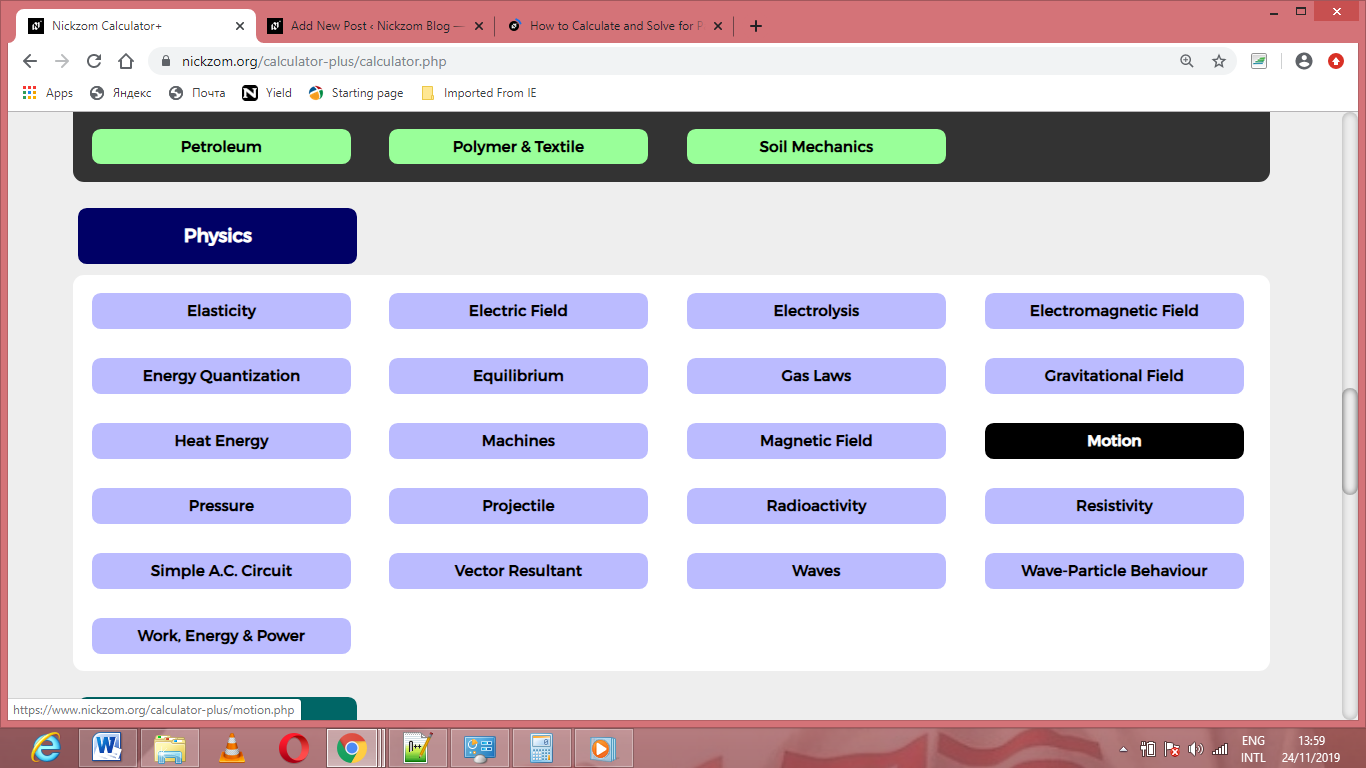Now, Click on Distance Covered under Motion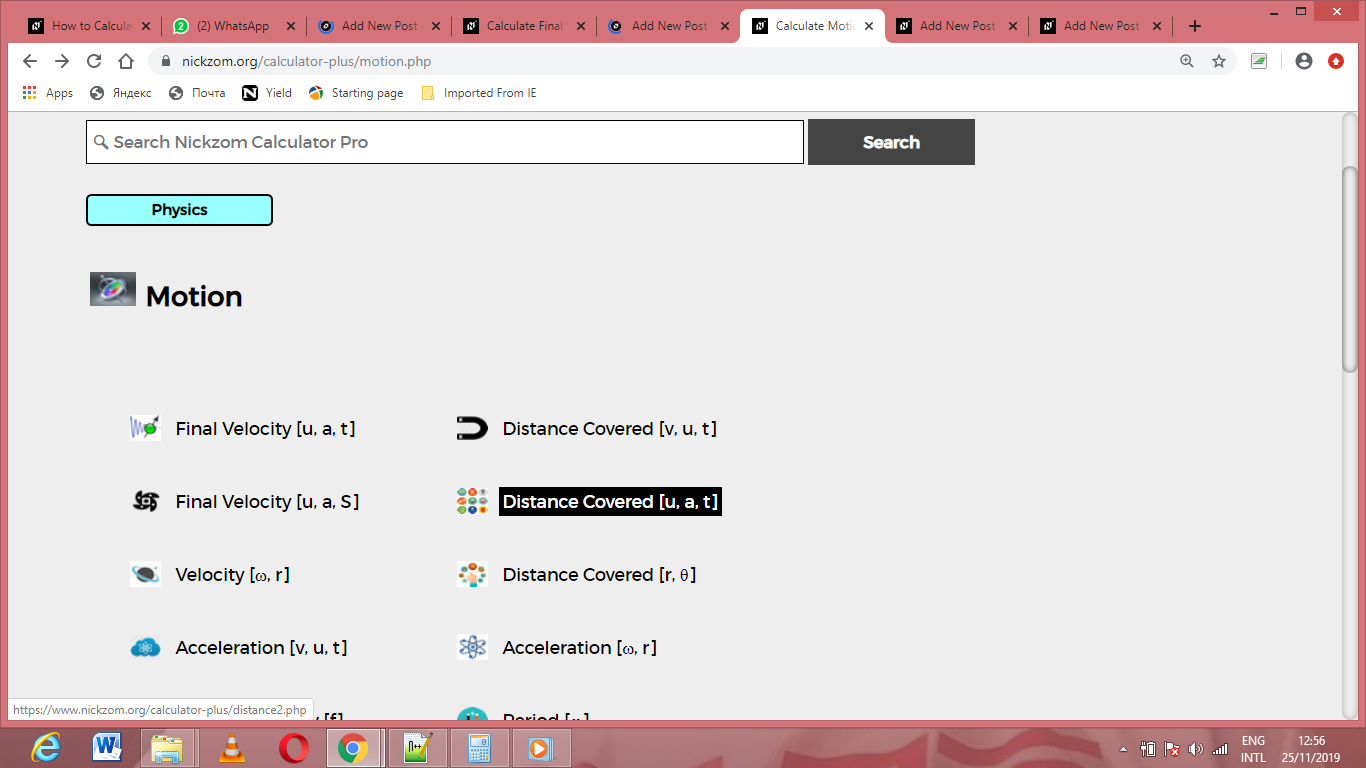The screenshot below displays the page or activity to enter your values, to get the answer for the distance covered according to the respective parameters which are the initial velocity (u), acceleration (a) and time (t).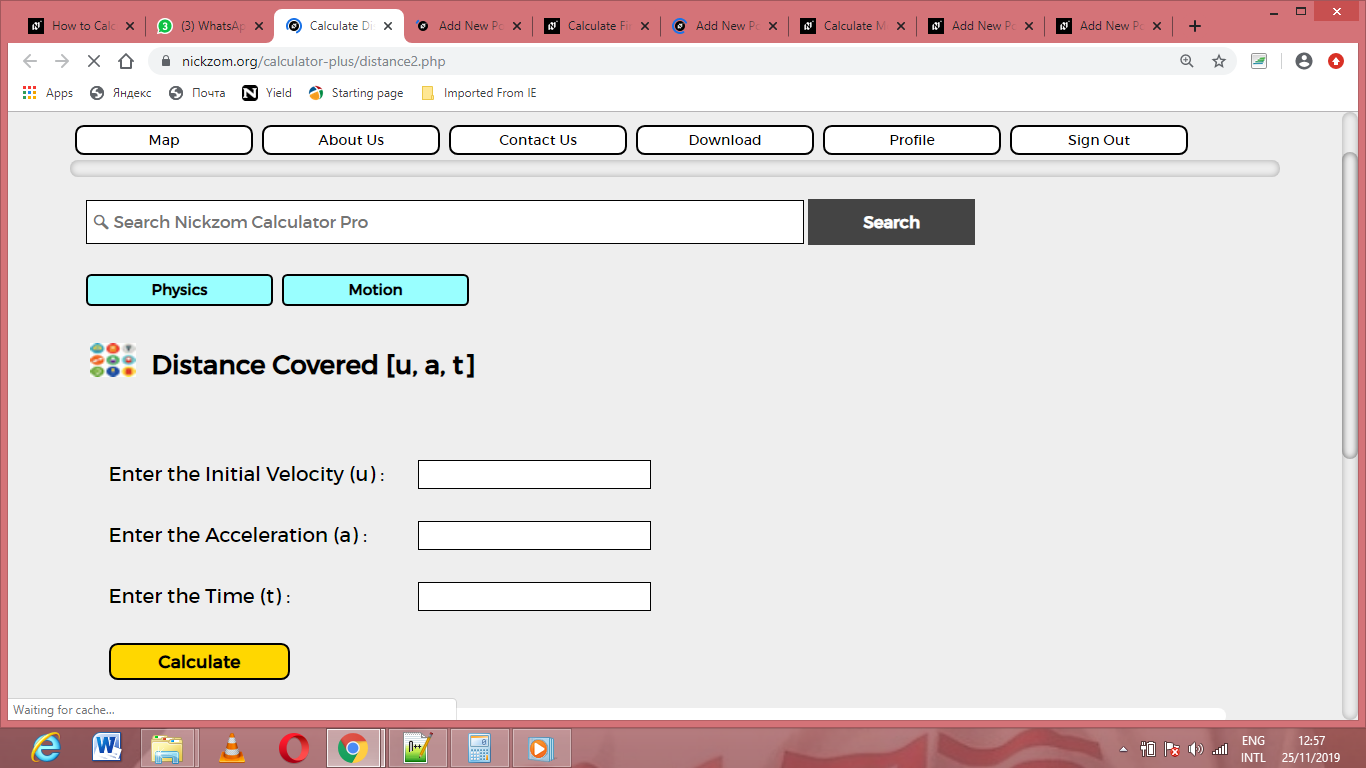Now, enter the values appropriately and accordingly for the parameters as required by the initial velocity (u) is 21, acceleration (a) is 10 and time (t) is 15.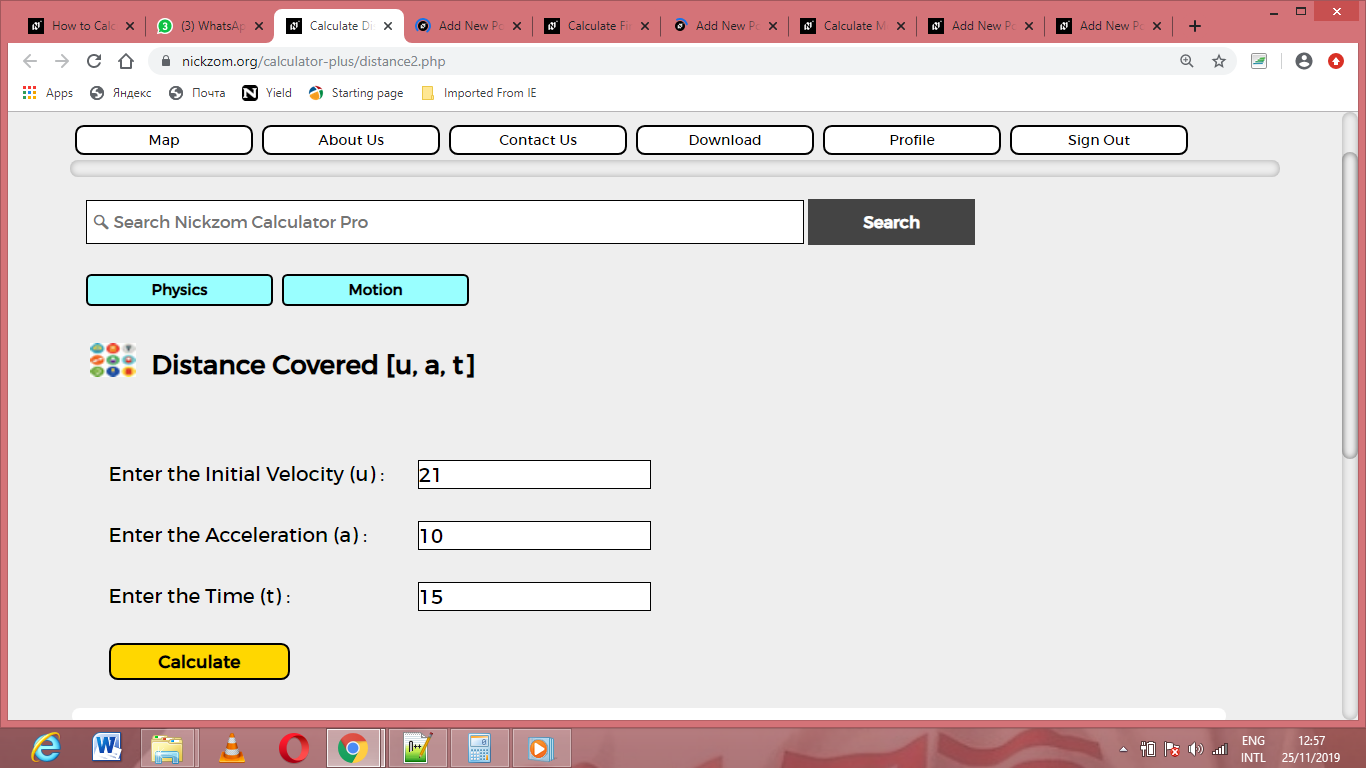Finally, Click on Calculate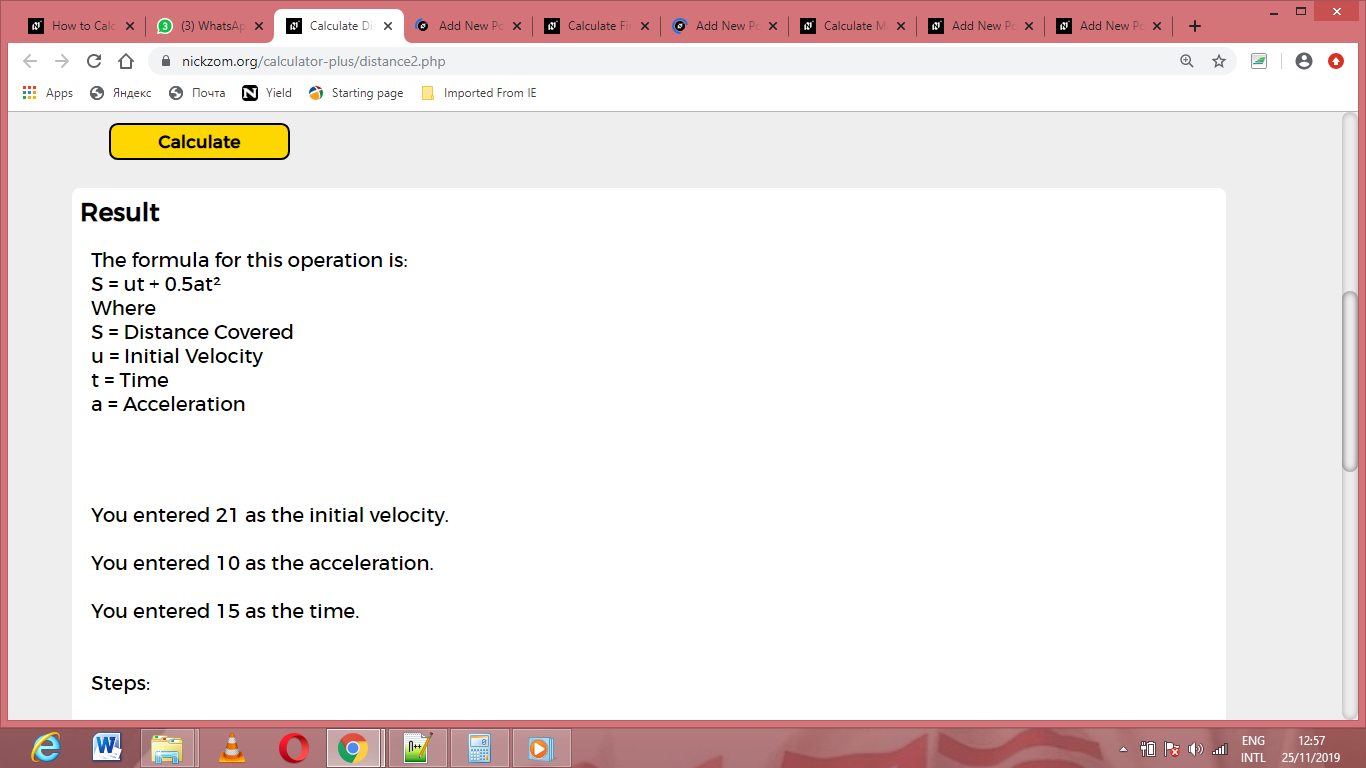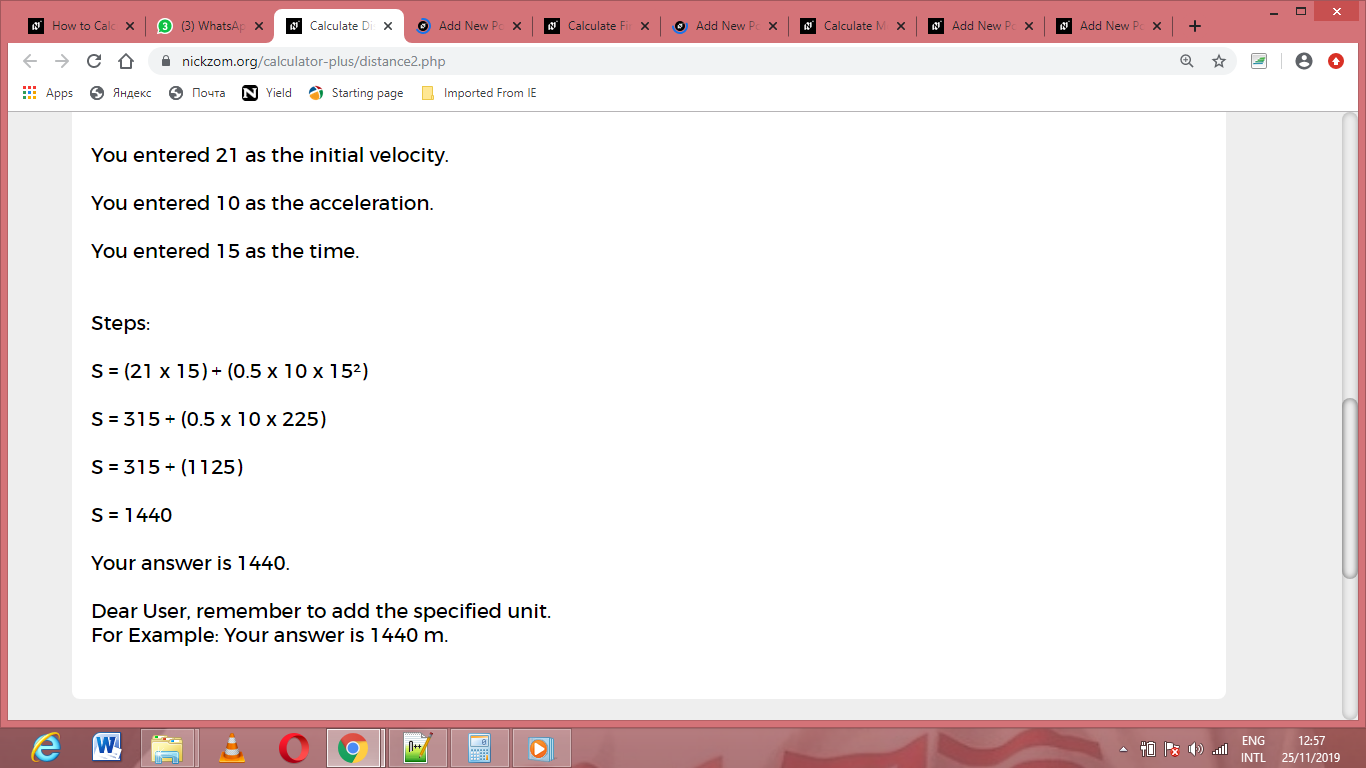As you can see from the screenshot above, Nickzom Calculator– The Calculator Encyclopedia solves for the distance covered and presents the formula, workings and steps too.